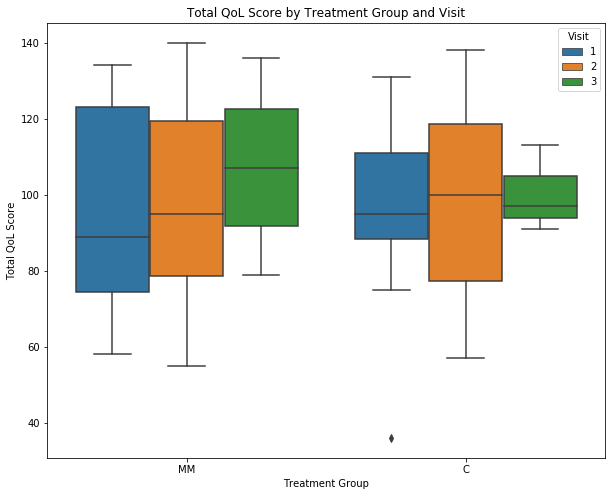# Cognitive Study (Second Update)¶

Feb. 1, 2019

## Part 1 - Data Cleaning¶

In :
import pandas as pd
import math
import numpy as np
import xlrd
import matplotlib.pyplot as plt
import seaborn as sns


Reading the excel file into Python. Note there are three sheets.

In :
Reg = pd.read_excel("cog3.xlsx", sheet_name="Registration")
Qol = pd.read_excel("cog3.xlsx", sheet_name="QOL score CMW")


First we clean the registration tab. We identify each patient with a PIN number, and each patient has a maximum of three visits.

In :
reg = Reg.sort_values(by='PIN').reset_index(drop=True)
reg = reg[['Visit', 'Age', 'Education',
'Gender', 'Handedness', 'Race',
'Ethnicity', 'Sequence', 'Disease']]

Out:
Visit Age Education Gender Handedness Race Ethnicity Sequence Disease
0 1 52 22 2 1 1 1 D MM
1 2 52 22 2 1 1 1 B MM
2 3 53 22 2 1 1 1 A MM
3 1 39 21 2 1 2 1 D C
4 2 40 21 2 1 2 1 C C

The cog variable contains information about the cognition scores of all patients. The Assessment Name variable agrees with the Visit variable in the previous part.

In :
cog = (Cog
.groupby(['PIN', 'Assessment Name'], sort=True)
.agg('mean')
.reset_index(drop=True)[['Fully-Corrected T-score']]
.rename(columns={'Fully-Corrected T-score' : 'Cog_Score'}))

Out:
Cog_Score
0 51.50
1 50.50
2 56.25
3 48.00
4 57.25

The qol variable contains data for the quality of life scores. We need to change the scaling of the scores so that we have the higher scores corresponding to more positive situation. According to the information provided in the QOL Interpretation section, we modify all the scores as follows.

In :
qol = Qol.fillna(np.nan)
qol.loc[:,'GP1':'GP6'] = qol.loc[:,'GP1':'GP6'].apply(lambda x: 4-x)
qol.loc[:,'GE1'] = qol.loc[:,'GE1'].apply(lambda x: 4-x)
qol.loc[:,'GE3':'GE6'] = qol.loc[:,'GP3':'GP6'].apply(lambda x: 4-x)
qol.loc[:,'P2':'HI7'] = qol.loc[:,'P2':'HI7'].apply(lambda x: 4-x)
qol.loc[:,'Physical_Score'] = qol.loc[:,'GP1':'GP7'].sum(axis=1)
qol.loc[:,'Social_Score'] = qol.loc[:,'GS1':'GS7'].sum(axis=1)
qol.loc[:,'Emotional_Score'] = qol.loc[:,'GE1':'GE6'].sum(axis=1)
qol.loc[:,'Functional_Score'] = qol.loc[:,'GF1':'GF7'].sum(axis=1)
qol.loc[:,'Total_Score'] = qol.loc[:,['Physical_Score', 'Social_Score',
'Emotional_Score', 'Functional_Score',
qol = qol.sort_values(by=['R#', 'Visit#'])
qol = qol[['Physical_Score', 'Social_Score', 'Emotional_Score',
qol = qol.dropna().reset_index(drop=True).loc[:72,]

Out:
Physical_Score Social_Score Emotional_Score Functional_Score Additional_Score Total_Score
0 18.0 28.0 5.0 18.0 38.0 107.0
1 21.0 27.0 3.0 27.0 45.0 123.0
2 23.0 27.0 8.0 28.0 50.0 136.0
3 18.0 17.0 4.0 18.0 38.0 95.0
4 23.0 27.0 8.0 26.0 51.0 135.0

Before merging, we check that reg, cog, and qol all have the same dimension.

In :
print('reg has dimension {}'.format(reg.shape))
print('cog has dimension {}'.format(cog.shape))
print('qol has dimension {}'.format(qol.shape))

reg has dimension (73, 9)
cog has dimension (73, 1)
qol has dimension (73, 6)

In :
df = pd.concat([reg, cog, qol], axis=1)


In this update, visit 2 gets a lot of new data. This is shown from the countplot below.

In :
plt.rcParams['figure.figsize'] = (10, 8)
ax = sns.countplot(df['Visit'].astype(str), data=df)
ax.set(xlabel='Visit', ylabel='Count',title="Count by Visit")
plt.savefig('fig1.jpeg', dpi=1200)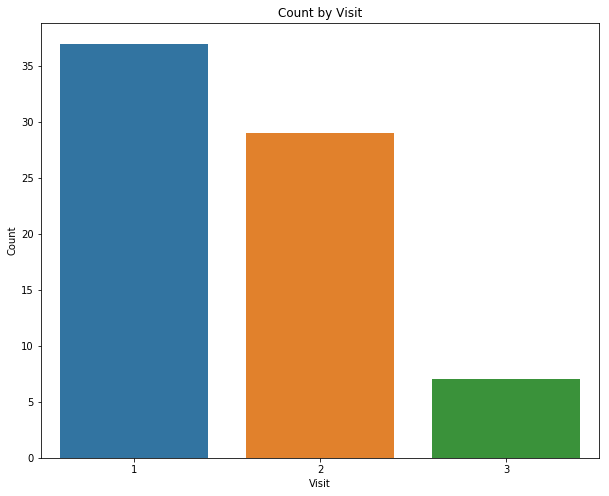## Part 2 - Exploratory Data Analysis¶

### 2.1   Plotting cognitive scores and total QOL scores stratified by disease (MM vs Control).

In :
ax = sns.lmplot(x='Cog_Score', y='Total_Score', hue='Disease', data=df, height=8)
ax.set(xlabel='Cog Score', ylabel='Total QoL Score',title="Cog Score vs Total QoL Score")
plt.savefig('fig2.jpeg', dpi=1200)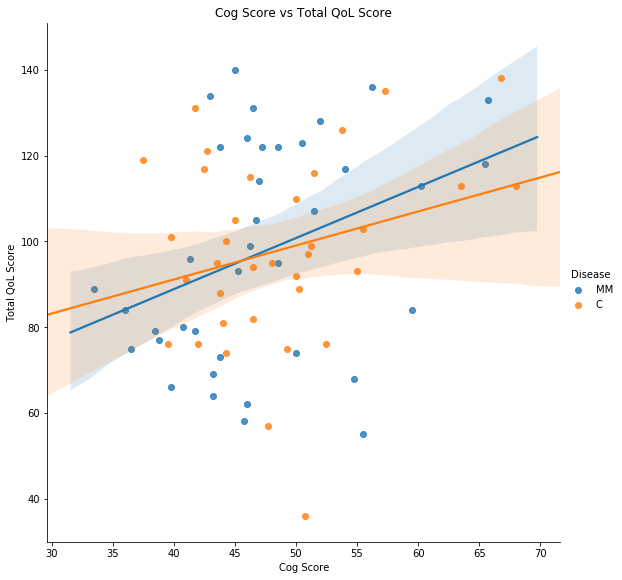It looks like that among MM patients, the cog score is inversely related to the QOL scores. For the control the relationship is opposite. This makes sense.

### 2.2   Does sequence affect cognitive scores?

In :
ax = sns.boxplot(x ='Sequence', y='Cog_Score', data=df)
ax.set(xlabel='Sequence', ylabel='Cog Score',title="Cog Score by Sequence")
plt.savefig('fig3.jpeg', dpi=1200)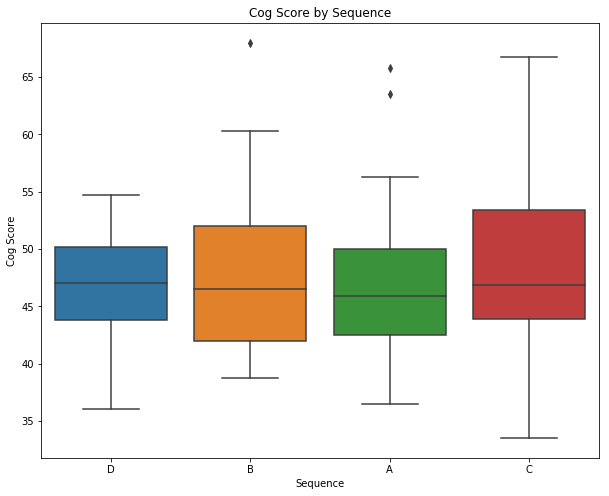Looks like that sequence A gives higher score. This is just a minor observation.

### 2.3   Is gender a significant factor?

In :
ax = sns.countplot(df['Gender'].astype(str), data=df)
ax.set(xlabel='Gender', ylabel='Count',title="Count by Gender")
plt.savefig('fig4.jpeg', dpi=1200)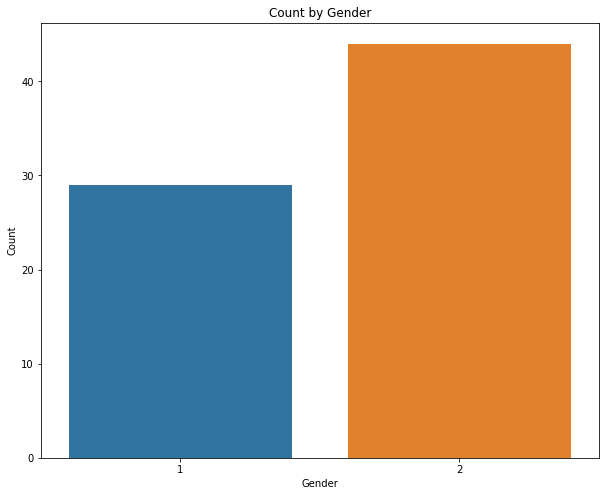In :
ax = sns.boxplot(x = 'Gender', y='Cog_Score', data=df)
ax.set(xlabel='Gender', ylabel='Cog Score',title="Cog Score by Gender")
plt.savefig('fig5.jpeg', dpi=1200)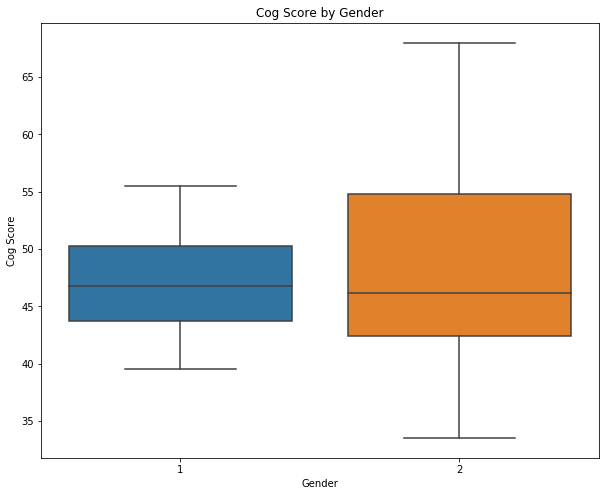In :
ax = sns.boxplot(x = 'Gender', y='Cog_Score', hue='Disease', data=df)
ax.set(xlabel='Gender', ylabel='Cog Score',title="Cog Score by Gender and Treatment Group")
plt.savefig('fig6.jpeg', dpi=1200)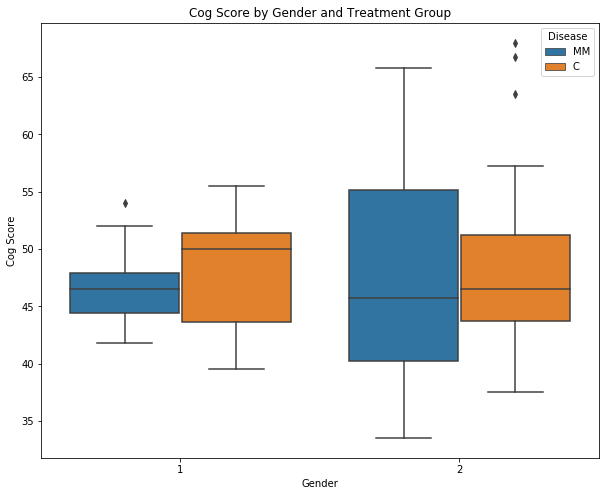In :
ax = sns.boxplot(x = 'Gender', y='Total_Score', data=df)
ax.set(xlabel='Gender', ylabel='Total QoL Score',title="Total QoL Score by Gender")
plt.savefig('fig7.jpeg', dpi=1200)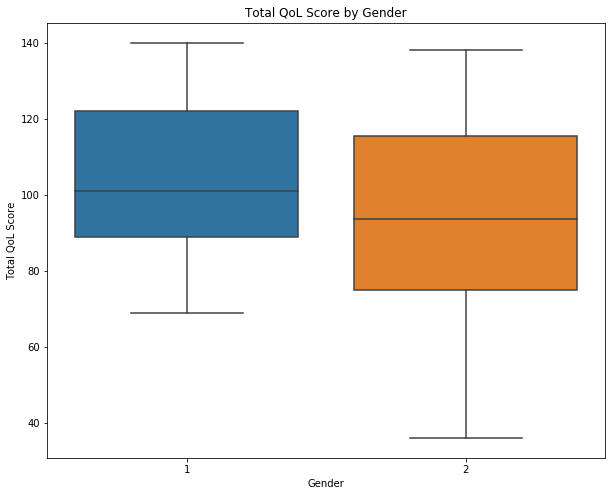It appears that gender is not a very significant factor.

### 2.4   Is there a difference between MM patients and control?

In :
ax = sns.countplot(df['Disease'].astype(str), data=df)
ax.set(xlabel='Treatment Group', ylabel='Count',title="Count by Treatment Group")
plt.savefig('fig8.jpeg', dpi=1200)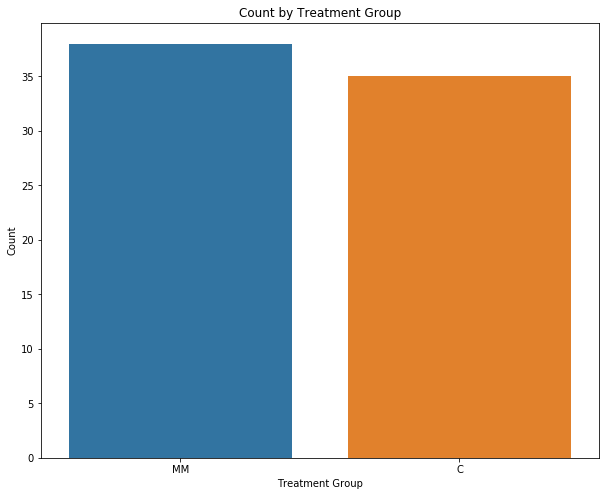In :
ax = sns.boxplot(x = 'Disease', y='Cog_Score', data=df)
ax.set(xlabel='Treatment Group', ylabel='Cog Score',title="Cog Score by Treatment Group")
plt.savefig('fig9.jpeg', dpi=1200)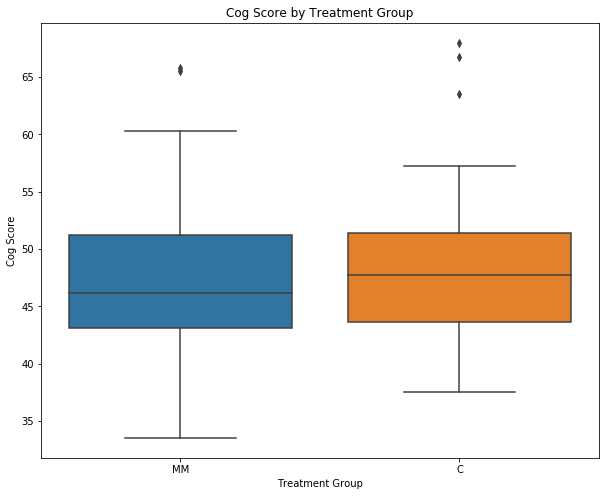In :
ax = sns.boxplot(x='Disease', y='Cog_Score', hue='Visit', data=df)
ax.set(xlabel='Treatment Group', ylabel='Cog Score',title="Cog Score by Treatment Group and Visit")
plt.savefig('fig10.jpeg', dpi=1200)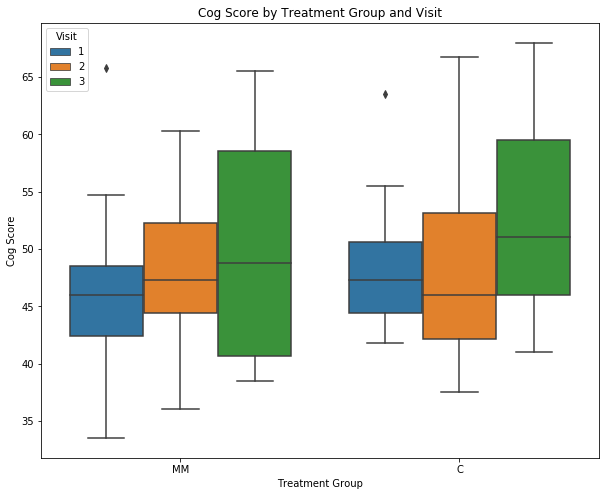Here we see the trend reverses from the previous two versions. Among the MM patients, we see a slight increase in cog score, while in the control, the score trends remain constant.

### 2.5   Statistical Analysis for 2.4

The slight difference observed above is not statistically significant, as shown below.

In :
visit1_MM = df[(df['Disease']=='MM') & (df["Visit"]==1)]["Cog_Score"]
visit2_MM = df[(df['Disease']=='MM') & (df["Visit"]==2)]["Cog_Score"]

visit1_C = df[(df['Disease']=='C') & (df["Visit"]==1)]["Cog_Score"]
visit2_C = df[(df['Disease']=='C') & (df["Visit"]==2)]["Cog_Score"]

print("Among the MM group, there are {} first visits, with mean equal to {}.".format(len(visit1_MM),np.mean(visit1_MM)))
print("Among the MM group, there are {} second visits, with mean equal to {}.".format(len(visit2_MM), np.mean(visit2_MM)))
print("Among the C group, there are {} first visits, with mean equal to {}.".format(len(visit1_C), np.mean(visit1_C)))
print("Among the C group, there are {} second visits, with mean equal to {}.".format(len(visit2_C), np.mean(visit2_C)))

Among the MM group, there are 19 first visits, with mean equal to 45.93421052631579.
Among the MM group, there are 15 second visits, with mean equal to 48.25.
Among the C group, there are 18 first visits, with mean equal to 48.416666666666664.
Among the C group, there are 14 second visits, with mean equal to 47.94642857142857.

In :
from scipy.stats import ttest_ind as ttest

t_MM, p_MM = ttest(visit1_MM, visit2_MM)
t_C, p_C = ttest(visit1_C, visit2_C)

print("Comparing cog scores between two visits among the MM group, the p-value is {}.".format(p_MM))
print("Comparing cog scores between two visits among the MM group, the p-value is {}.".format(p_C))

Comparing cog scores between two visits among the MM group, the p-value is 0.3453694345791114.
Comparing cog scores between two visits among the MM group, the p-value is 0.845954642439394.


### 2.6   Quality of life score by treatment group and visit

In :
ax = sns.boxplot(x = 'Disease', y='Physical_Score', hue='Visit', data=df)
ax.set(xlabel='Treatment Group', ylabel='Physical Score',title="Physical Score by Treatment Group and Visit")
plt.savefig('fig11.jpeg', dpi=1200)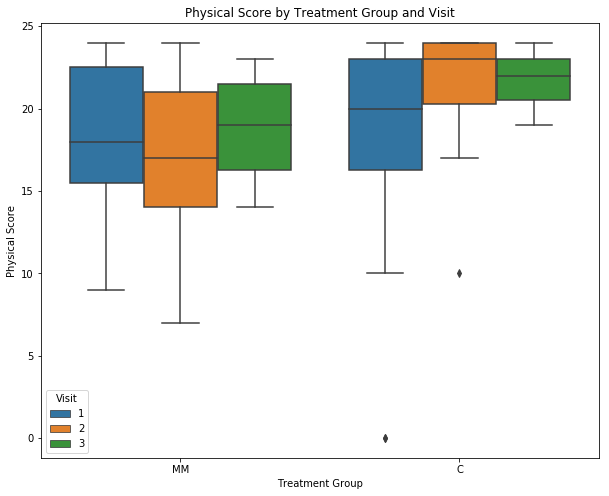In :
ax = sns.boxplot(x = 'Disease', y='Social_Score', data=df)
ax.set(xlabel='Treatment Group', ylabel='Social Score',title="Social Score by Treatment Group")
plt.savefig('fig12.jpeg', dpi=1200)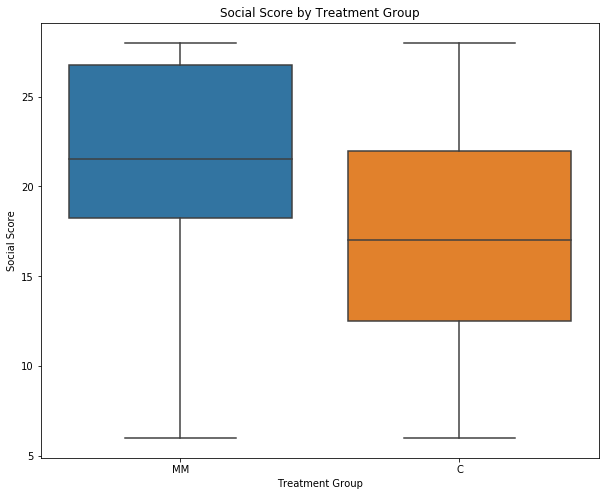In :
ax = sns.boxplot(x = 'Disease', y='Social_Score', hue='Visit', data=df)
ax.set(xlabel='Treatment Group', ylabel='Social Score',title="Social Score by Treatment Group and Visit")
plt.savefig('fig13.jpeg', dpi=1200)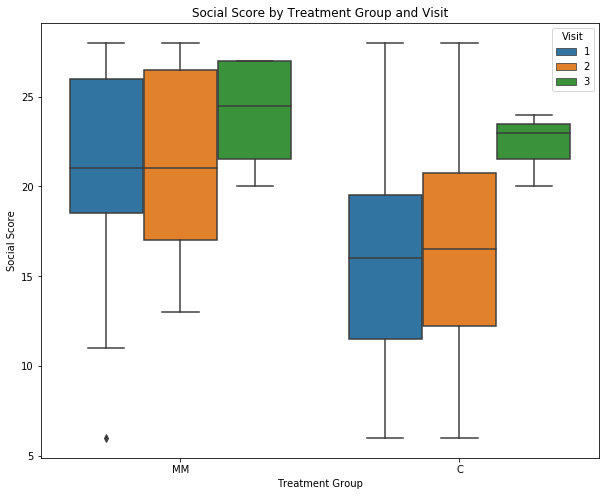In :
ax = sns.boxplot(x = 'Disease', y='Emotional_Score', data=df)
ax.set(xlabel='Treatment Group', ylabel='Emotional Score',title="Emotional Score by Treatment Group")
plt.savefig('fig14.jpeg', dpi=1200)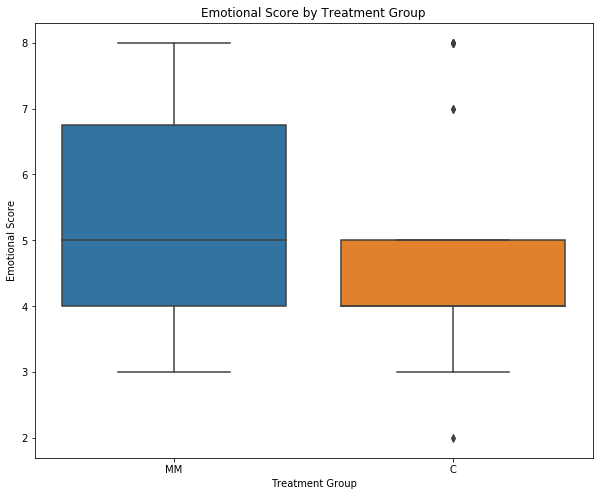In :
ax = sns.boxplot(x = 'Disease', y='Emotional_Score', hue='Visit', data=df)
ax.set(xlabel='Treatment Group', ylabel='Emotional Score',title="Emotional Score by Treatment Group and Visit")
plt.savefig('fig15.jpeg', dpi=1200)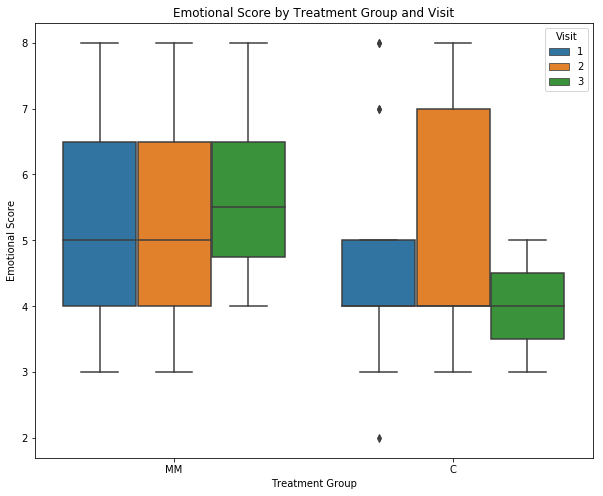In :
ax = sns.boxplot(x = 'Disease', y='Functional_Score', data=df)
ax.set(xlabel='Treatment Group', ylabel='Functional Score',title="Functional Score by Treatment Group")
plt.savefig('fig16.jpeg', dpi=1200)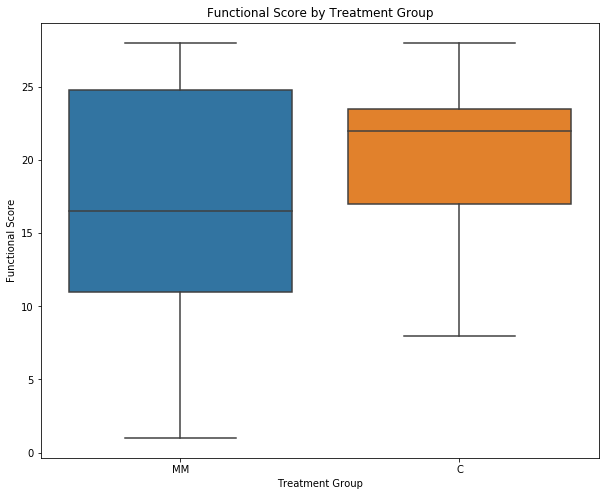In :
ax = sns.boxplot(x = 'Disease', y='Functional_Score', hue='Visit', data=df)
ax.set(xlabel='Treatment Group', ylabel='Functional Score',title="Functional Score by Treatment Group and Visit")
plt.savefig('fig17.jpeg', dpi=1200)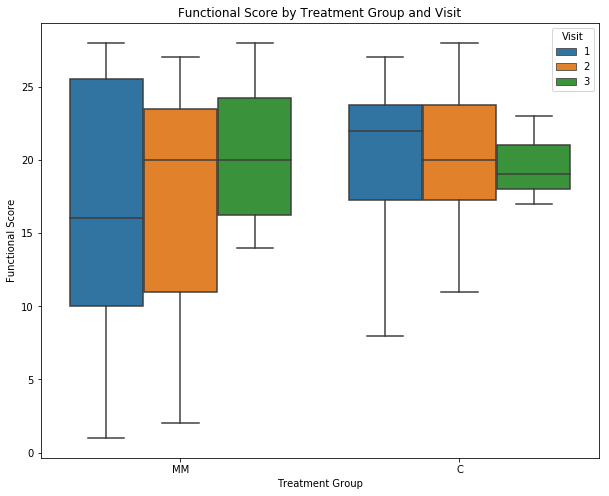In :
ax = sns.boxplot(x = 'Disease', y='Additional_Score', data=df)
plt.savefig('fig18.jpeg', dpi=1200)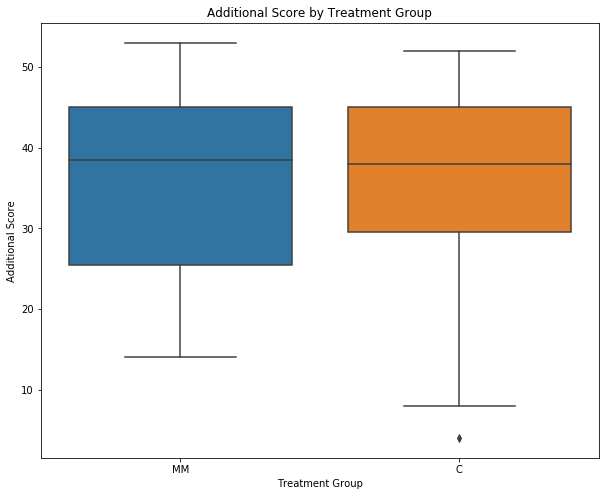In :
ax = sns.boxplot(x = 'Disease', y='Additional_Score', hue='Visit', data=df)
plt.savefig('fig19.jpeg', dpi=1200)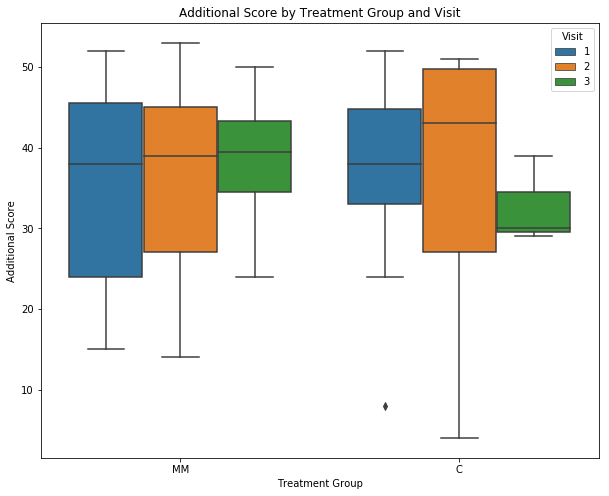In :
ax = sns.boxplot(x = 'Disease', y='Total_Score', data=df)
ax.set(xlabel='Treatment Group', ylabel='Total QoL Score',title="Total QoL Score by Treatment Group")
plt.savefig('fig20.jpeg', dpi=1200)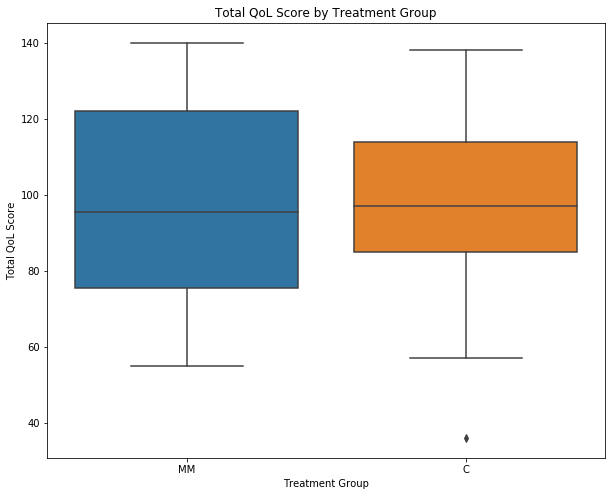In :
ax = sns.boxplot(x = 'Disease', y='Total_Score', hue='Visit', data=df)
ax.set(xlabel='Treatment Group', ylabel='Total QoL Score',title="Total QoL Score by Treatment Group and Visit")
plt.savefig('fig21.jpeg', dpi=1200)